# Chemical Reactions An Introduction Chapter 6 Chemical Reactions

• Slides: 26Chemical Reactions: An Introduction Chapter 6Chemical Reactions • Reactions involve chemical changes in matter resulting in new substances • Reactions involve rearrangement and exchange of atoms to produce new molecules – Elements are not transmuted during a reaction Reactants ProductsEvidence of Chemical Reactions • a chemical change occurs when new substances are made • visual clues (permanent) – color change, precipitate formation, gas bubbles, flames, heat release, cooling, light • other clues – new odor, permanent new state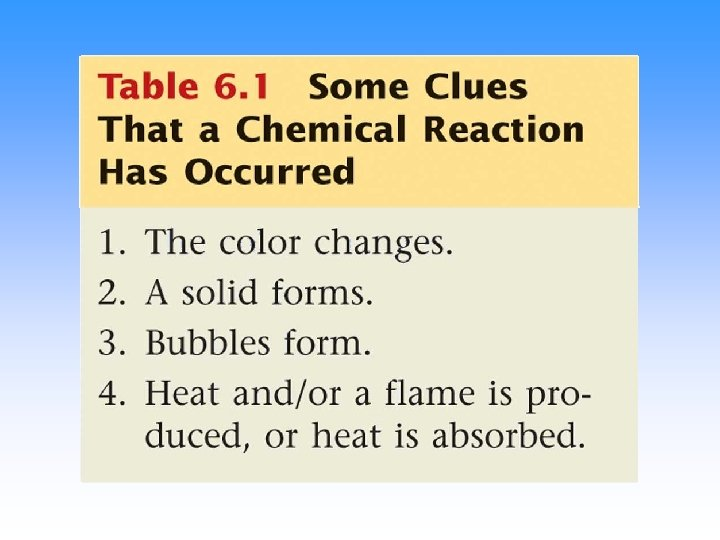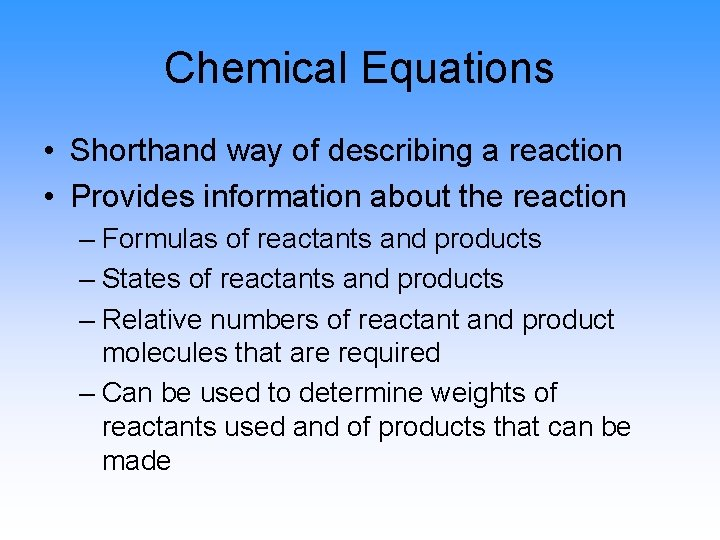Chemical Equations • Shorthand way of describing a reaction • Provides information about the reaction – Formulas of reactants and products – States of reactants and products – Relative numbers of reactant and product molecules that are required – Can be used to determine weights of reactants used and of products that can be made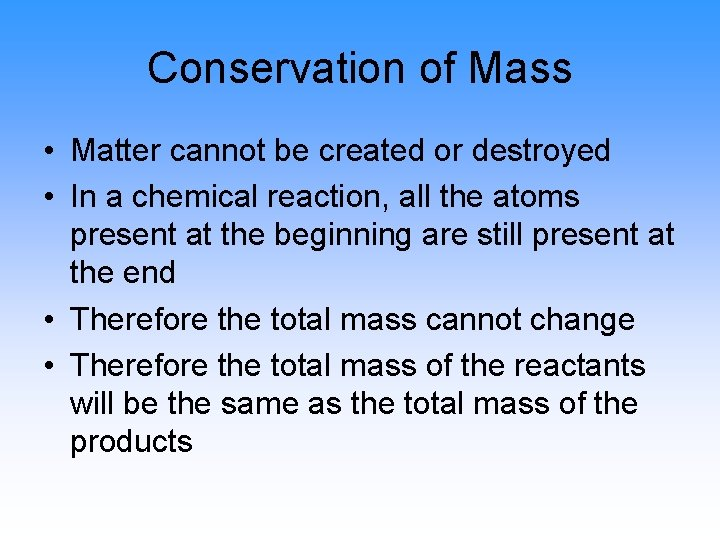Conservation of Mass • Matter cannot be created or destroyed • In a chemical reaction, all the atoms present at the beginning are still present at the end • Therefore the total mass cannot change • Therefore the total mass of the reactants will be the same as the total mass of the products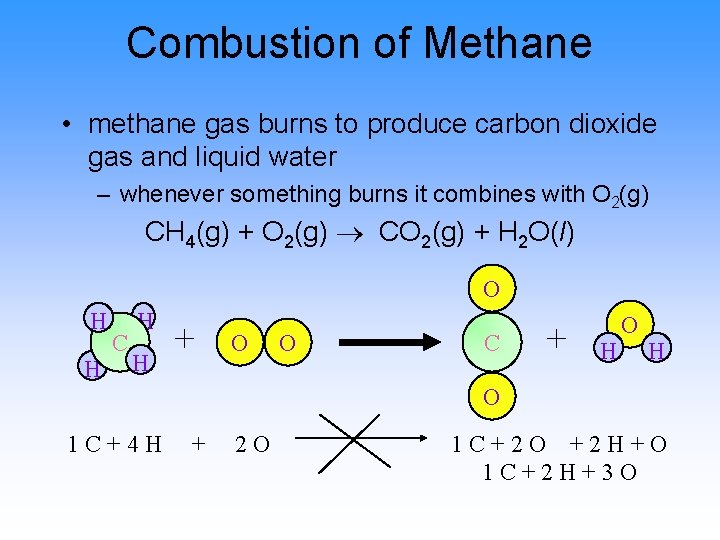Combustion of Methane • methane gas burns to produce carbon dioxide gas and liquid water – whenever something burns it combines with O 2(g) CH 4(g) + O 2(g) CO 2(g) + H 2 O(l) O H H C H H + O O C + H O 1 C+4 H + 2 O 1 C+2 O +2 H+O 1 C+2 H+3 O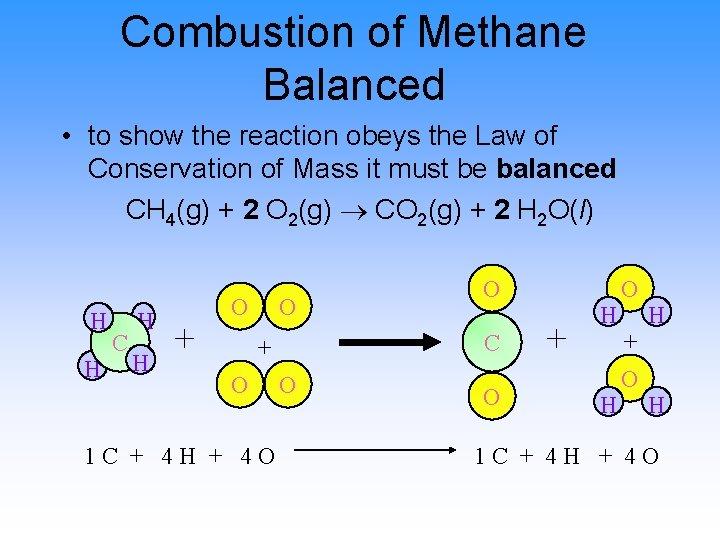Combustion of Methane Balanced • to show the reaction obeys the Law of Conservation of Mass it must be balanced CH 4(g) + 2 O 2(g) CO 2(g) + 2 H 2 O(l) H H C H H + O O C + O 1 C + 4 H + 4 O O + H H O + O H H 1 C + 4 H + 4 O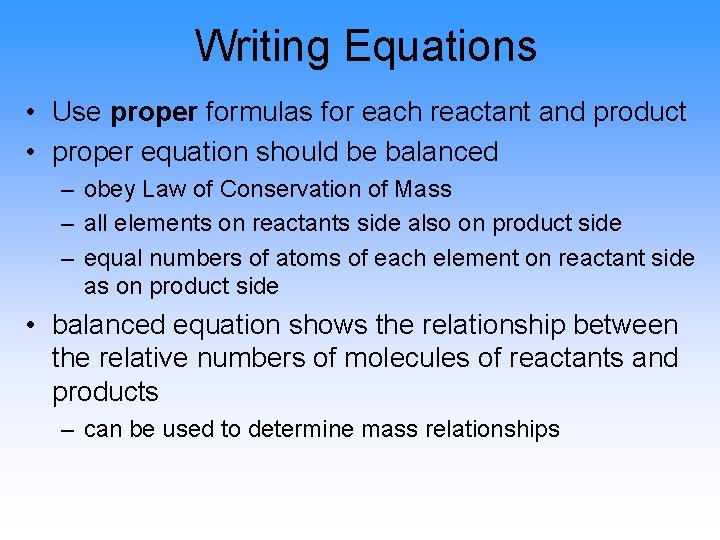Writing Equations • Use proper formulas for each reactant and product • proper equation should be balanced – obey Law of Conservation of Mass – all elements on reactants side also on product side – equal numbers of atoms of each element on reactant side as on product side • balanced equation shows the relationship between the relative numbers of molecules of reactants and products – can be used to determine mass relationships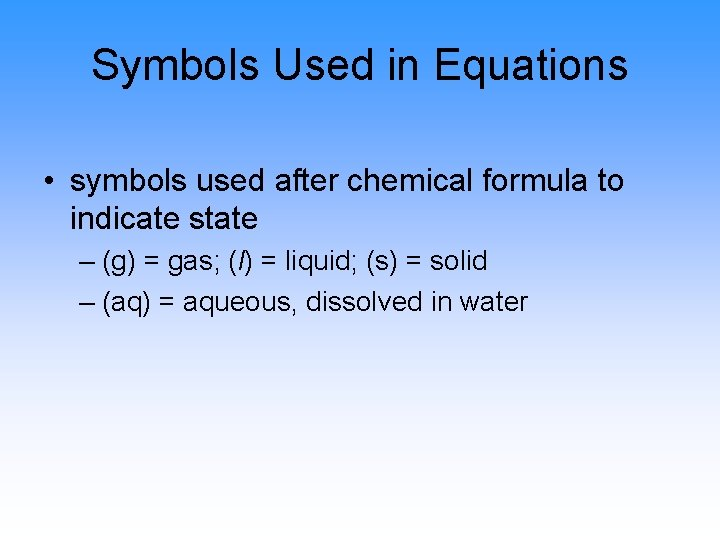Symbols Used in Equations • symbols used after chemical formula to indicate state – (g) = gas; (l) = liquid; (s) = solid – (aq) = aqueous, dissolved in water• Sample – Recognizing Reactants and Products when magnesium metal burns in air it produces a white, powdery compound magnesium oxide – burning in air means reacting with O 2 – Metals are solids, except for Hg which is liquid ¬ write the equation in words – identify the state of each chemical magnesium(s) + oxygen(g) magnesium oxide(s) write the equation in formulas – identify diatomic elements – identify polyatomic ions – determine formulas Mg(s) + O 2(g) Mg. O(s)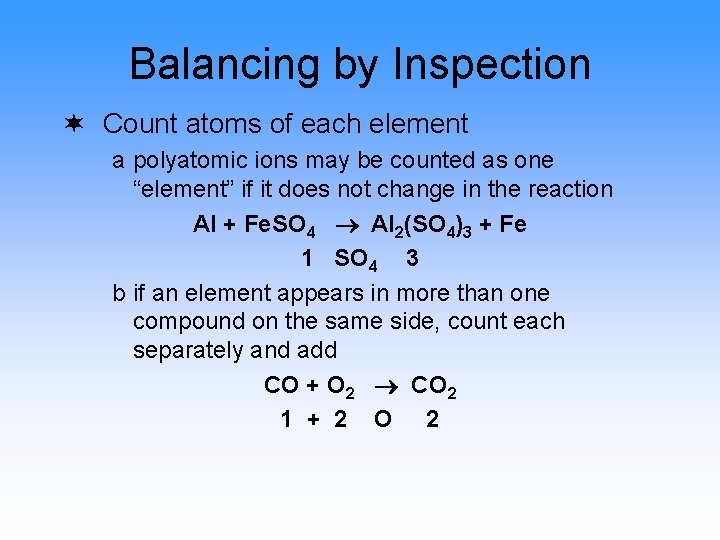Balancing by Inspection ¬ Count atoms of each element a polyatomic ions may be counted as one “element” if it does not change in the reaction Al + Fe. SO 4 Al 2(SO 4)3 + Fe 1 SO 4 3 b if an element appears in more than one compound on the same side, count each separately and add CO + O 2 CO 2 1 + 2 O 2Balancing by Inspection • Pick an element to balance • avoid elements from 1 b • • Find Least Common Multiple and factors needed to make both sides equal Use factors as coefficients in equation • if already a coefficient then multiply by new factor • Recount and Repeat until balancedA bombardier beetle defending itself.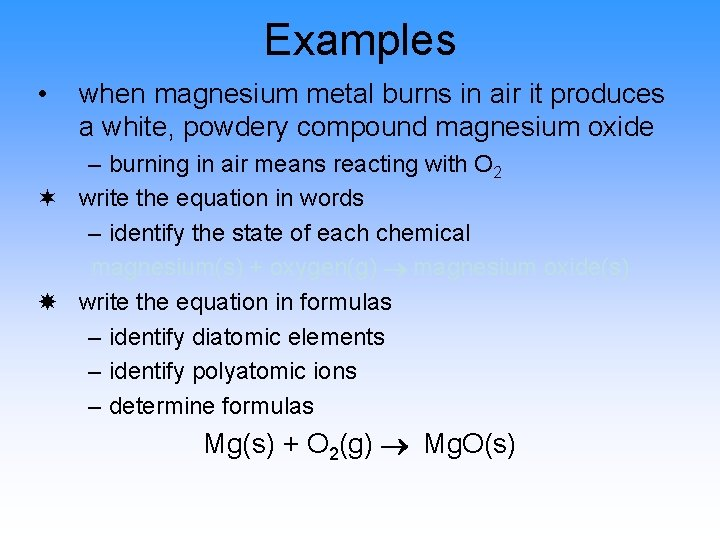Examples • when magnesium metal burns in air it produces a white, powdery compound magnesium oxide – burning in air means reacting with O 2 ¬ write the equation in words – identify the state of each chemical magnesium(s) + oxygen(g) magnesium oxide(s) write the equation in formulas – identify diatomic elements – identify polyatomic ions – determine formulas Mg(s) + O 2(g) Mg. O(s)Examples • when magnesium metal burns in air it produces a white, powdery compound magnesium oxide – burning in air means reacting with O 2 ® count the number of atoms of on each side – count polyatomic groups as one “element” if on both sides – split count of element if in more than one compound on one side Mg(s) + O 2(g) Mg. O(s) 1 Mg 1 2 O 1Examples • ¯ ° when magnesium metal burns in air it produces a white, powdery compound magnesium oxide – burning in air means reacting with O 2 pick an element to balance – avoid element in multiple compounds find least common multiple of both sides & multiply each side by factor so it equals LCM Mg(s) + O 2(g) Mg. O(s) 1 Mg 1 1 x 2 O 1 x 2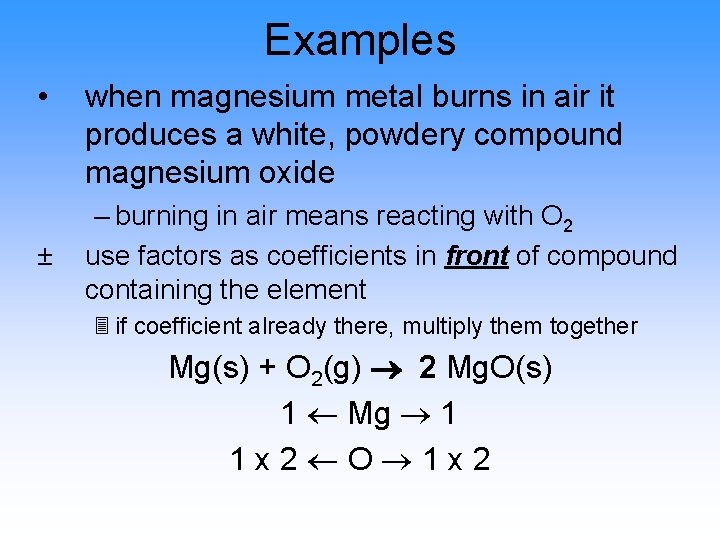Examples • ± when magnesium metal burns in air it produces a white, powdery compound magnesium oxide – burning in air means reacting with O 2 use factors as coefficients in front of compound containing the element 3 if coefficient already there, multiply them together Mg(s) + O 2(g) 2 Mg. O(s) 1 Mg 1 1 x 2 O 1 x 2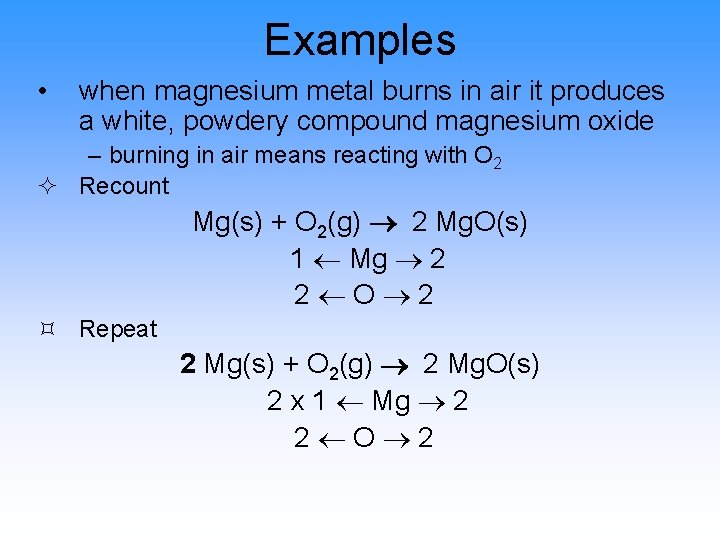Examples • when magnesium metal burns in air it produces a white, powdery compound magnesium oxide – burning in air means reacting with O 2 ² Recount Mg(s) + O 2(g) 2 Mg. O(s) 1 Mg 2 2 O 2 ³ Repeat 2 Mg(s) + O 2(g) 2 Mg. O(s) 2 x 1 Mg 2 2 O 2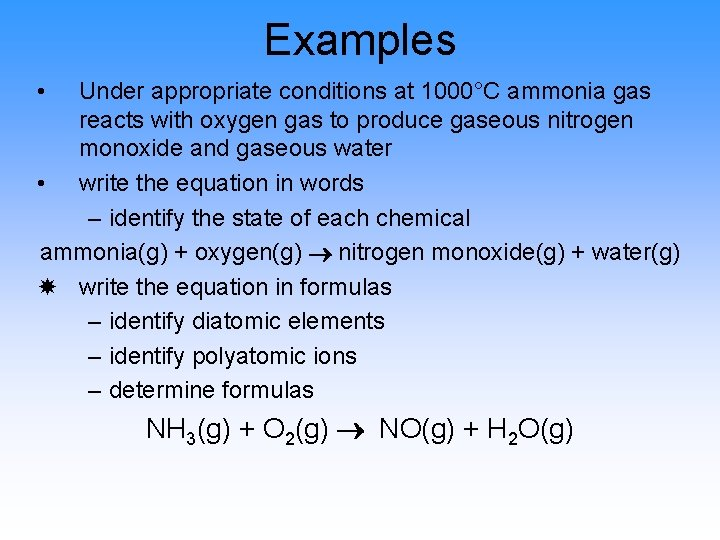Examples • Under appropriate conditions at 1000°C ammonia gas reacts with oxygen gas to produce gaseous nitrogen monoxide and gaseous water • write the equation in words – identify the state of each chemical ammonia(g) + oxygen(g) nitrogen monoxide(g) + water(g) write the equation in formulas – identify diatomic elements – identify polyatomic ions – determine formulas NH 3(g) + O 2(g) NO(g) + H 2 O(g)Examples • Under appropriate conditions at 1000°C ammonia gas reacts with oxygen gas to produce gaseous nitrogen monoxide and gaseous water ® count the number of atoms of on each side – count polyatomic groups as one “element” if on both sides – split count of element if in more than one compound on one side NH 3(g) + O 2(g) NO(g) + H 2 O(g) 1 N 1 3 H 2 2 O 1+1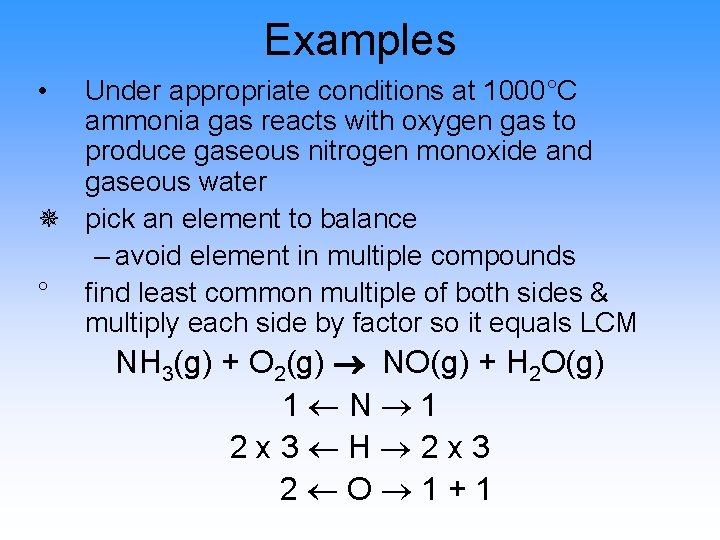Examples • Under appropriate conditions at 1000°C ammonia gas reacts with oxygen gas to produce gaseous nitrogen monoxide and gaseous water ¯ pick an element to balance – avoid element in multiple compounds ° find least common multiple of both sides & multiply each side by factor so it equals LCM NH 3(g) + O 2(g) NO(g) + H 2 O(g) 1 N 1 2 x 3 H 2 x 3 2 O 1+1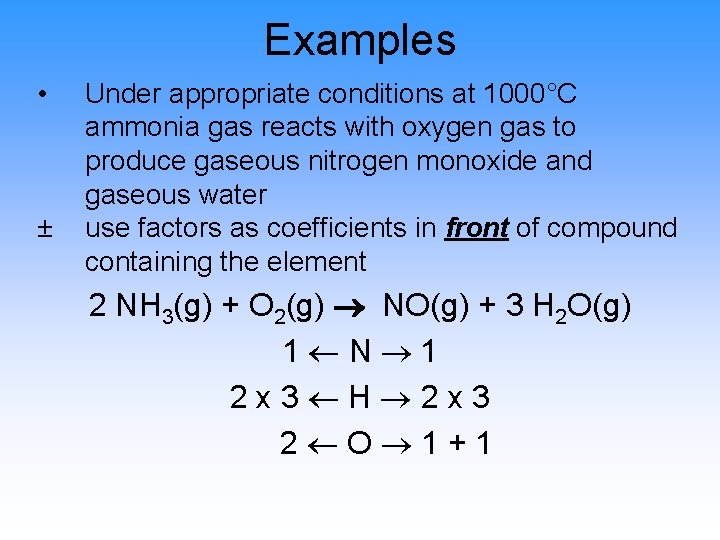Examples • ± Under appropriate conditions at 1000°C ammonia gas reacts with oxygen gas to produce gaseous nitrogen monoxide and gaseous water use factors as coefficients in front of compound containing the element 2 NH 3(g) + O 2(g) NO(g) + 3 H 2 O(g) 1 N 1 2 x 3 H 2 x 3 2 O 1+1Examples • Under appropriate conditions at 1000°C ammonia gas reacts with oxygen gas to produce gaseous nitrogen monoxide and gaseous water ² Recount 2 NH 3(g) + O 2(g) NO(g) + 3 H 2 O(g) 2 N 1 6 H 6 2 O 1+3 ³ Repeat 2 NH 3(g) + O 2(g) 2 NO(g) + 3 H 2 O(g) 2 N 1 x 2 6 H 6 2 O 1+3Examples • ´ Under appropriate conditions at 1000°C ammonia gas reacts with oxygen gas to produce gaseous nitrogen monoxide and gaseous water Recount 2 NH 3(g) + O 2(g) 2 NO(g) + 3 H 2 O(g) 2 N 2 6 H 6 2 O 2+3Examples • Under appropriate conditions at 1000°C ammonia gas reacts with oxygen gas to produce gaseous nitrogen monoxide and gaseous water µ Repeat – A trick of the trade, when you are forced to attack an element that is in 3 or more compounds – find where it is uncombined. You can find a factor to make it any amount you want, even if that factor is a fraction! – We want to make the O on the left equal 5, therefore we will multiply it by 2. 5 2 NH 3(g) + 2. 5 O 2(g) 2 NO(g) + 3 H 2 O(g) 2 N 2 6 H 6 2. 5 x 2 O 2 + 3